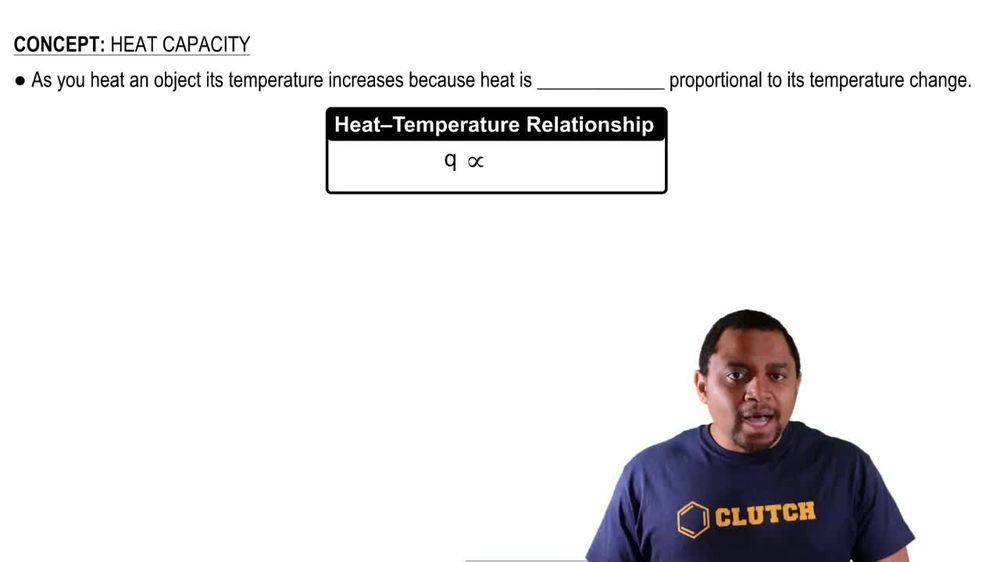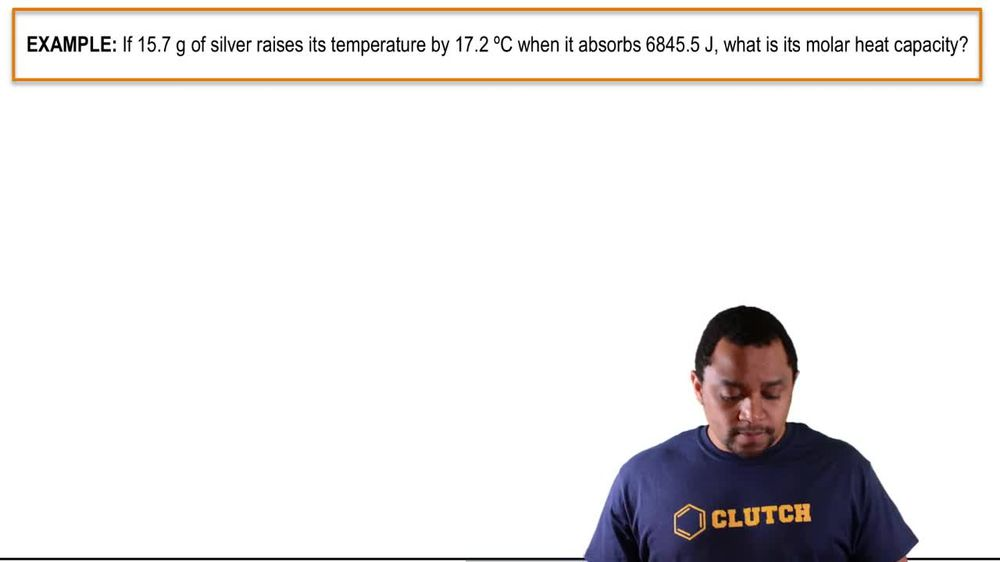Start typing, then use the up and down arrows to select an option from the list.
3:21 minutes
Problem 145
Textbook Question

# We said in Section 9.1 that the potential energy of water at the top of a dam or waterfall is converted into heat when the water dashes against rocks at the bottom. The potential energy of the water at the top is equal to EP = mgh, where m is the mass of the water, g is the acceleration of the falling water due to gravity 1g = 9.81 m>s22, and h is the height of the water. Assuming that all the energy is converted to heat, calculate the temperature rise of the water in degrees Celsius after falling over California’s Yosemite Falls, a distance of 739 m. The specific heat of water is 4.18 J/(g·K).Verified Solution
This video solution was recommended by our tutors as helpful for the problem above.
154views

### Watch next

Master Heat Capacity with a bite sized video explanation from Jules Bruno

Start learning00:3100:3302:1901:4700:4402:08